Mathematics
Easy

Question

# The steps of a proof are shown.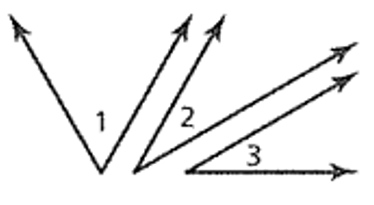Given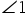is a complement of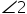.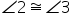Proveis a complement of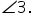What is reason 5? Statements Reasons 1. ∠1 is a complement of ∠2. 1. Given 2. ∠2 ≡ ∠3   3. m ∠ 1 + m ∠ 2 = 90°   4. m ∠ 2 = m ∠ 3   5. m ∠ 1 + m ∠ 3 = 90°   6. ∠1 is a complement of ∠3.

## Definition of complementary anglesSubstitution Property of EqualityDefinition of congruent anglesSubtraction Property of EqualityHint:

## The correct answer is: Substitution Property of Equality

### Here , we have to find that reason of step 5.Firstly , we have is ∠1 is complement of ∠2 and ∠2 ≅∠3And steps are, ∠1 is complement of ∠2 (given) ∠2 ≅∠3 (given) m∠1 + m ∠2 = 90 ( complementary angle) m ∠2 = m ∠3 ( both angle is congruent ) m ∠2 + m ∠3 = 90 ( substitution property ) ∠1 is complement of ∠3Therefore, the reason of step 5 is substitution property of equality.The correct answer is Substitution property of Equality.Or,Substitution Property of EqualityThe whole proof: Statements Reasons 1. ∠1 is a complement of ∠2. 1. Given 2. ∠2 ≡ ∠3 2. Given 3. m ∠ 1 + m ∠ 2 = 90° 3. Definition of complementary angles  4. m ∠ 2 = m ∠ 3 4. Definition of congruent angles 5. m ∠ 1 + m ∠ 3 = 90° 5. Substitution Property of Equality 6. ∠1 is a complement of ∠3. 6. Definition of complementary angles

In this question, we have to find the reason of step 5 which is substitution property. In substitution property of equality , if X = Y and X = Z then Y = Z.

### Related Questions to study#### With Turito Foundation.#### Get an Expert Advice From Turito.